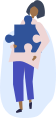Chemistry Homework Help

Get step-by-step solutions from expert tutors.

## How it works?Type, take a picture, or paste your question with all necessary details to ensure high-quality responses.### Get an answer in less than 24hrs

Once our tutors receive the question, they'll begin. You'll get an email once the solution is ready.### Get Notified

We will send you email and text notification to let you know when your question is answered.

### Browse Homework Solutions

12,927 solutions

Phase Diagram

#### Q. A phase diagram is a pressure-temperature graph that shows the rangers of temperature and pressure over which each phase is stable. The phase diagram is divided into three regions, each region representing one stable phase: solid, liquid, or gas. There are three boundary lines that separate the phases from one another. These boundary lines represent the equilibrium between two phases. the point at which the three boundary lines intersect is called the triple point. All three phases can exist in equilibrium with each other at this point. Select the image to explore the interactive activity that shows the phase diagram for CO2. Using the interactive diagram, you can observe what the phase of CO2 is at a particular temperature and pressure by moving the cursor to that point on the phase diagram. The animation also shows the type of movement experienced by CO2 molecules in a particular phase. You are given six samples of carbon dioxide (CO2) that are stored at different temperatures and at different pressures. Using the interactive activity showing the phase diagram of CO2, determine the phases of each of the samples and classify them according to which phase they are in. Keep in mind that the temperature and pressure are not to scale. Drag the appropriate items to their respective bins.

Solved • Jan 20, 2020

Phase Diagram

#### Q. The triple point of water is 0.0098 °C at 0.00603 atm (4.58 torr). At the triple point, ice, water, and water vapor exist in equilibrium with each other. Complete the following sentences to identify the process that ice, water, or water vapor may undergo if either the temperature or the pressure is increased. Match the words in the left column to the appropriate blanks in the sentences on the right. Make certain each sentence is complete before submitting your answer.

Solved • Jan 20, 2020

Density

#### Q. Student Z completes his/her calibration step for part 3 and finds an average massA to be 95.237 g ± 0.005 g. However, in his/her calculations, s/he mistakenly uses 85.237 g ± 0.005 g for massA. S/he uses the correct values for massB and mass cyl. Will the calculated density be too high, too low, or unchanged?

Solved • Jan 20, 2020

Phase Diagram

#### Q. A phase diagram is a temperature-pressure plot that summarizes the conditions under which a substance exists as a solid, liquid, or gas. The curves that separate the phases are known as phase boundaries. Each phase boundary represents the equilibrium between the phases on either side of the curve. Identify the components of the phase diagram of water. Drag the appropriate labels to their respective targets.

Solved • Jan 20, 2020

Entropy

#### Q. The enthalpy of vaporization of water at 100.0°C is 40.68 kJ/mol. What is the entropy change in the surroundings when one mole of water vapor condenses at 100.0°C in a large room maintained at a temperature of 25.00°C? A.−136.4 J/ K . D.+109.0J / K B.−109.0J/ K . E.+136.4 J / K . C.−40.68 J / K

Solved • Jan 20, 2020

Phase Diagram

#### Q. Point A to G are located on the phase diagram of water. Which of the following statements are correct regarding navigation from one point to another across the phase diagram? Check all that apply.

Solved • Jan 20, 2020

Phase Diagram

#### Q. A phase diagram is a temperature-pressure plot that summarizes the conditions under which a substance exists as a solid, liquid, or gas. The curves that separate the phases are known as phase boundaries. Each phase boundary represents the equilibrium between the phases on either side of the curve. Identify the components of the phase diagram of water. Drag the appropriate labels to their respective targets.

Solved • Jan 20, 2020